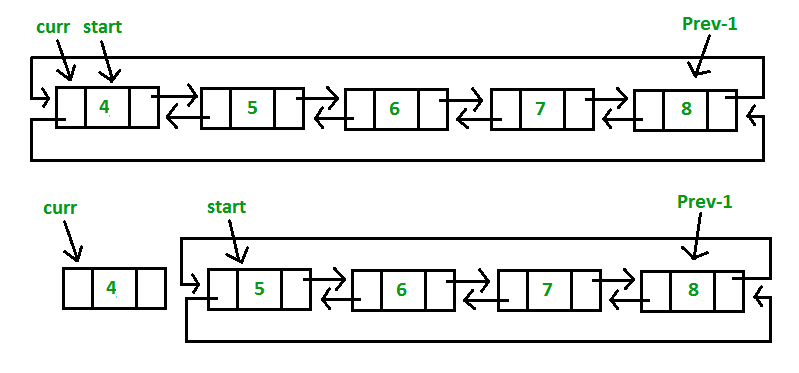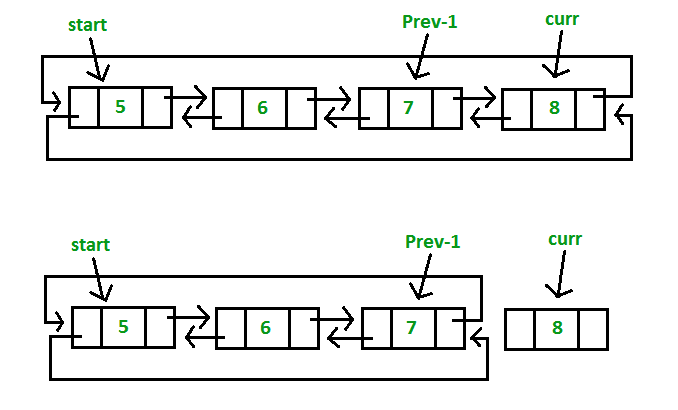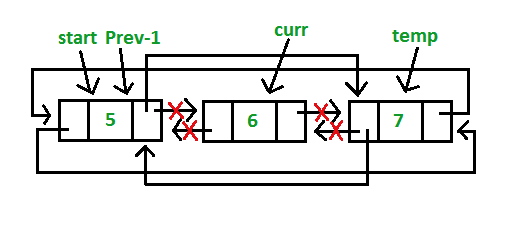# Doubly Circular Linked List | Set 2 (Deletion)

• Difficulty Level : Basic
• Last Updated : 23 Jun, 2022

We have discussed the doubly circular linked list introduction and its insertion.
Let us formulate the problem statement to understand the deletion process. Given a ‘key’, delete the first occurrence of this key in the circular doubly linked list.

Algorithm

Case 1: Empty List(start = NULL)

• If the list is empty, simply return it.

Case 2: The List initially contains some nodes, start points at the first node of the List

1. If the list is not empty, then we define two pointers curr and prev_1 and initialize the pointer curr points to the first node of the list, and prev_1 = NULL.
2. Traverse the list using the curr pointer to find the node to be deleted and before moving from curr to the next node, every time set prev_1 = curr.
3. If the node is found, check if it is the only node in the list. If yes, set start = NULL and free the node pointing by curr.
4. If the list has more than one node, check if it is the first node of the list. The condition to check this is (curr == start). If yes, then move prev_1 to the last node(prev_1 = start -> prev). After prev_1 reaches the last node, set start = start -> next and prev_1 -> next = start and start -> prev = prev_1. Free the node pointing by curr.
5. If curr is not the first node, we check if it is the last node in the list. The condition to check this is (curr -> next == start). If yes, set prev_1 -> next = start and start -> prev = prev_1. Free the node pointing by curr.
6. If the node to be deleted is neither the first node nor the last node, declare one more pointer temp and initialize the pointer temp points to the next of curr pointer (temp = curr->next). Now set, prev_1 -> next = temp and temp ->prev = prev_1. Free the node pointing by curr.
• If the given key(Say 4) matches with the first node of the list(Step 4):• If the given key(Say 8) matches with the last node of the list(Step 5):• If the given key(Say 6) matches with the middle node of the list(Step 6):## C++

 `// C++ program to delete a given key from` `// circular doubly linked list.` `#include ` `using` `namespace` `std;`   `// Structure of a Node` `struct` `Node {` `    ``int` `data;` `    ``struct` `Node* next;` `    ``struct` `Node* prev;` `};`   `// Function to insert node in the list` `void` `insert(``struct` `Node** start, ``int` `value)` `{` `    ``// If the list is empty, create a single node` `    ``// circular and doubly list` `    ``if` `(*start == NULL) {` `        ``struct` `Node* new_node = ``new` `Node;` `        ``new_node->data = value;` `        ``new_node->next = new_node->prev = new_node;` `        ``*start = new_node;` `        ``return``;` `    ``}`   `    ``// If list is not empty`   `    ``/* Find last node */` `    ``Node* last = (*start)->prev;`   `    ``// Create Node dynamically` `    ``struct` `Node* new_node = ``new` `Node;` `    ``new_node->data = value;`   `    ``// Start is going to be next of new_node` `    ``new_node->next = *start;`   `    ``// Make new node previous of start` `    ``(*start)->prev = new_node;`   `    ``// Make last previous of new node` `    ``new_node->prev = last;`   `    ``// Make new node next of old last` `    ``last->next = new_node;` `}`   `// Function to delete a given node from the list` `void` `deleteNode(``struct` `Node** start, ``int` `key)` `{` `    ``// If list is empty` `    ``if` `(*start == NULL)` `        ``return``;`   `    ``// Find the required node` `    ``// Declare two pointers and initialize them` `    ``struct` `Node *curr = *start, *prev_1 = NULL;` `    ``while` `(curr->data != key) {` `        ``// If node is not present in the list` `        ``if` `(curr->next == *start) {` `            ``printf``(``"\nList doesn't have node with value = %d"``, key);` `            ``return``;` `        ``}`   `        ``prev_1 = curr;` `        ``curr = curr->next;` `    ``}`   `    ``// Check if node is the only node in list` `    ``if` `(curr->next == *start && prev_1 == NULL) {` `        ``(*start) = NULL;` `        ``free``(curr);` `        ``return``;` `    ``}`   `    ``// If list has more than one node,` `    ``// check if it is the first node` `    ``if` `(curr == *start) {` `        ``// Move prev_1 to last node` `        ``prev_1 = (*start)->prev;`   `        ``// Move start ahead` `        ``*start = (*start)->next;`   `        ``// Adjust the pointers of prev_1 and start node` `        ``prev_1->next = *start;` `        ``(*start)->prev = prev_1;` `        ``free``(curr);` `    ``}`   `    ``// check if it is the last node` `    ``else` `if` `(curr->next == *start) {` `        ``// Adjust the pointers of prev_1 and start node` `        ``prev_1->next = *start;` `        ``(*start)->prev = prev_1;` `        ``free``(curr);` `    ``}` `    ``else` `{` `        ``// create new pointer, points to next of curr node` `        ``struct` `Node* temp = curr->next;`   `        ``// Adjust the pointers of prev_1 and temp node` `        ``prev_1->next = temp;` `        ``temp->prev = prev_1;` `        ``free``(curr);` `    ``}` `}`   `// Function to display list elements` `void` `display(``struct` `Node* start)` `{` `    ``struct` `Node* temp = start;`   `    ``while` `(temp->next != start) {` `        ``printf``(``"%d "``, temp->data);` `        ``temp = temp->next;` `    ``}` `    ``printf``(``"%d "``, temp->data);` `}`   `// Driver program to test above functions` `int` `main()` `{` `    ``// Start with the empty list` `    ``struct` `Node* start = NULL;`   `    ``// Created linked list will be 4->5->6->7->8` `    ``insert(&start, 4);` `    ``insert(&start, 5);` `    ``insert(&start, 6);` `    ``insert(&start, 7);` `    ``insert(&start, 8);`   `    ``printf``(``"List Before Deletion: "``);` `    ``display(start);`   `    ``// Delete the node which is not present in list` `    ``deleteNode(&start, 9);` `    ``printf``(``"\nList After Deletion: "``);` `    ``display(start);`   `    ``// Delete the first node` `    ``deleteNode(&start, 4);` `    ``printf``(``"\nList After Deleting %d: "``, 4);` `    ``display(start);`   `    ``// Delete the last node` `    ``deleteNode(&start, 8);` `    ``printf``(``"\nList After Deleting %d: "``, 8);` `    ``display(start);`   `    ``// Delete the middle node` `    ``deleteNode(&start, 6);` `    ``printf``(``"\nList After Deleting %d: "``, 6);` `    ``display(start);`   `    ``return` `0;` `}`

## Java

 `// Java program to delete a given key from` `// circular doubly linked list.` `class` `GFG {`   `    ``// structure of a Node` `    ``static` `class` `Node {` `        ``int` `data;` `        ``Node next;` `        ``Node prev;` `    ``};`   `    ``// Function to insert node in the list` `    ``static` `Node insert(Node start, ``int` `value)` `    ``{` `        ``// If the list is empty, create a single node` `        ``// circular and doubly list` `        ``if` `(start == ``null``) {` `            ``Node new_node = ``new` `Node();` `            ``new_node.data = value;` `            ``new_node.next = new_node.prev = new_node;` `            ``start = new_node;` `            ``return` `start;` `        ``}`   `        ``// If list is not empty`   `        ``// Find last node /` `        ``Node last = (start).prev;`   `        ``// Create Node dynamically` `        ``Node new_node = ``new` `Node();` `        ``new_node.data = value;`   `        ``// Start is going to be next of new_node` `        ``new_node.next = start;`   `        ``// Make new node previous of start` `        ``(start).prev = new_node;`   `        ``// Make last previous of new node` `        ``new_node.prev = last;`   `        ``// Make new node next of old last` `        ``last.next = new_node;` `        ``return` `start;` `    ``}`   `    ``// Function to delete a given node from the list` `    ``static` `Node deleteNode(Node start, ``int` `key)` `    ``{` `        ``// If list is empty` `        ``if` `(start == ``null``)` `            ``return` `null``;`   `        ``// Find the required node` `        ``// Declare two pointers and initialize them` `        ``Node curr = start, prev_1 = ``null``;` `        ``while` `(curr.data != key) {` `            ``// If node is not present in the list` `            ``if` `(curr.next == start) {` `                ``System.out.printf(``"\nList doesn't have node with value = %d"``, key);` `                ``return` `start;` `            ``}`   `            ``prev_1 = curr;` `            ``curr = curr.next;` `        ``}`   `        ``// Check if node is the only node in list` `        ``if` `(curr.next == start && prev_1 == ``null``) {` `            ``(start) = ``null``;` `            ``return` `start;` `        ``}`   `        ``// If list has more than one node,` `        ``// check if it is the first node` `        ``if` `(curr == start) {` `            ``// Move prev_1 to last node` `            ``prev_1 = (start).prev;`   `            ``// Move start ahead` `            ``start = (start).next;`   `            ``// Adjust the pointers of prev_1 and start node` `            ``prev_1.next = start;` `            ``(start).prev = prev_1;` `        ``}`   `        ``// check if it is the last node` `        ``else` `if` `(curr.next == start) {` `            ``// Adjust the pointers of prev_1 and start node` `            ``prev_1.next = start;` `            ``(start).prev = prev_1;` `        ``}` `        ``else` `{` `            ``// create new pointer, points to next of curr node` `            ``Node temp = curr.next;`   `            ``// Adjust the pointers of prev_1 and temp node` `            ``prev_1.next = temp;` `            ``temp.prev = prev_1;` `        ``}` `        ``return` `start;` `    ``}`   `    ``// Function to display list elements` `    ``static` `void` `display(Node start)` `    ``{` `        ``Node temp = start;`   `        ``while` `(temp.next != start) {` `            ``System.out.printf(``"%d "``, temp.data);` `            ``temp = temp.next;` `        ``}` `        ``System.out.printf(``"%d "``, temp.data);` `    ``}`   `    ``// Driver program to test above functions` `    ``public` `static` `void` `main(String args[])` `    ``{` `        ``// Start with the empty list` `        ``Node start = ``null``;`   `        ``// Created linked list will be 4.5.6.7.8` `        ``start = insert(start, ``4``);` `        ``start = insert(start, ``5``);` `        ``start = insert(start, ``6``);` `        ``start = insert(start, ``7``);` `        ``start = insert(start, ``8``);`   `        ``System.out.printf(``"List Before Deletion: "``);` `        ``display(start);`   `        ``// Delete the node which is not present in list` `        ``start = deleteNode(start, ``9``);` `        ``System.out.printf(``"\nList After Deletion: "``);` `        ``display(start);`   `        ``// Delete the first node` `        ``start = deleteNode(start, ``4``);` `        ``System.out.printf(``"\nList After Deleting %d: "``, ``4``);` `        ``display(start);`   `        ``// Delete the last node` `        ``start = deleteNode(start, ``8``);` `        ``System.out.printf(``"\nList After Deleting %d: "``, ``8``);` `        ``display(start);`   `        ``// Delete the middle node` `        ``start = deleteNode(start, ``6``);` `        ``System.out.printf(``"\nList After Deleting %d: "``, ``6``);` `        ``display(start);` `    ``}` `}`   `// This code is contributed by Arnab Kundu`

## Python3

 `# Python3 program to delete a given key from` `# circular doubly linked list.`   `# structure of a node of linked list ` `class` `Node: ` `    ``def` `__init__(``self``, data): ` `        ``self``.data ``=` `data ` `        ``self``.``next` `=` `None` `        ``self``.prev ``=` `None`   `def` `insert( start, value):` `    `  `    ``# If the list is empty, create a single node` `    ``# circular and doubly list` `    ``if` `(start ``=``=` `None``): ` `        ``new_node ``=` `Node(``0``)` `        ``new_node.data ``=` `value` `        ``new_node.``next` `=` `new_node.prev ``=` `new_node` `        ``start ``=` `new_node` `        ``return` `start` `        `  `    ``# If list is not empty`   `    ``# Find last node /` `    ``last ``=` `(start).prev`   `    ``# Create Node dynamically` `    ``new_node ``=` `Node(``0``)` `    ``new_node.data ``=` `value`   `    ``# Start is going to be next of new_node` `    ``new_node.``next` `=` `start`   `    ``# Make new node previous of start` `    ``(start).prev ``=` `new_node`   `    ``# Make last previous of new node` `    ``new_node.prev ``=` `last`   `    ``# Make new node next of old last` `    ``last.``next` `=` `new_node` `    ``return` `start` `    `  `# Function to delete a given node` `# from the list` `def` `deleteNode(start, key):` `    `  `    ``# If list is empty` `    ``if` `(start ``=``=` `None``):` `        ``return` `None`   `    ``# Find the required node` `    ``# Declare two pointers and initialize them` `    ``curr ``=` `start` `    ``prev_1 ``=` `None` `    ``while` `(curr.data !``=` `key) :` `        `  `        ``# If node is not present in the list` `        ``if` `(curr.``next` `=``=` `start) :` `            ``print` `(``"List doesn't have node"``, ` `                       ``"with value = "``, key)` `            ``return` `start` `            `  `        ``prev_1 ``=` `curr` `        ``curr ``=` `curr.``next` `        `  `    ``# Check if node is the only node in list` `    ``if` `(curr.``next` `=``=` `start ``and` `prev_1 ``=``=` `None``) :` `        ``(start) ``=` `None` `        ``return` `start` `        `  `    ``# If list has more than one node,` `    ``# check if it is the first node` `    ``if` `(curr ``=``=` `start) :` `        `  `        ``# Move prev_1 to last node` `        ``prev_1 ``=` `(start).prev`   `        ``# Move start ahead` `        ``start ``=` `(start).``next`   `        ``# Adjust the pointers of prev_1 ` `        ``# and start node` `        ``prev_1.``next` `=` `start` `        ``(start).prev ``=` `prev_1` `        `  `    ``# check if it is the last node` `    ``elif` `(curr.``next` `=``=` `start) :` `        `  `        ``# Adjust the pointers of prev_1 ` `        ``# and start node` `        ``prev_1.``next` `=` `start` `        ``(start).prev ``=` `prev_1` `        `  `    ``else` `:` `        `  `        ``# create new pointer,` `        ``# points to next of curr node` `        ``temp ``=` `curr.``next`   `        ``# Adjust the pointers of prev_1` `        ``# and temp node` `        ``prev_1.``next` `=` `temp` `        ``temp.prev ``=` `prev_1` `        `  `    ``return` `start` `    `  `# Function to display list elements` `def` `display(start):` `    `  `    ``temp ``=` `start`   `    ``while` `(temp.``next` `!``=` `start) :` `        ``print` `(temp.data, end ``=` `" "``) ` `        ``temp ``=` `temp.``next` `        `  `    ``print` `(temp.data)` `    `  `# Driver Code ` `if` `__name__``=``=``'__main__'``: ` `    `  `    ``# Start with the empty list` `    ``start ``=` `None`   `    ``# Created linked list will be 4.5.6.7.8` `    ``start ``=` `insert(start, ``4``)` `    ``start ``=` `insert(start, ``5``)` `    ``start ``=` `insert(start, ``6``)` `    ``start ``=` `insert(start, ``7``)` `    ``start ``=` `insert(start, ``8``)`   `    ``print` `(``"List Before Deletion: "``)` `    ``display(start)`   `    ``# Delete the node which is not present in list` `    ``start ``=` `deleteNode(start, ``9``)` `    ``print` `(``"List After Deletion: "``)` `    ``display(start)`   `    ``# Delete the first node` `    ``start ``=` `deleteNode(start, ``4``)` `    ``print` `(``"List After Deleting"``, ``4``)` `    ``display(start)`   `    ``# Delete the last node` `    ``start ``=` `deleteNode(start, ``8``)` `    ``print` `(``"List After Deleting "``, ``8``)` `    ``display(start)`   `    ``# Delete the middle node` `    ``start ``=` `deleteNode(start, ``6``)` `    ``print` `(``"List After Deleting "``, ``6``)` `    ``display(start)` `    `  `# This code is contributed by Arnab Kundu`

## C#

 `// C# program to delete a given key from` `// circular doubly linked list.` `using` `System;`   `class` `GFG {`   `    ``// structure of a Node` `    ``public` `class` `Node {` `        ``public` `int` `data;` `        ``public` `Node next;` `        ``public` `Node prev;` `    ``};`   `    ``// Function to insert node in the list` `    ``static` `Node insert(Node start, ``int` `value)` `    ``{` `        ``// If the list is empty, create a single node` `        ``// circular and doubly list` `        ``Node new_node = ``new` `Node();` `        ``if` `(start == ``null``) {`   `            ``new_node.data = value;` `            ``new_node.next = new_node.prev = new_node;` `            ``start = new_node;` `            ``return` `start;` `        ``}`   `        ``// If list is not empty`   `        ``// Find last node /` `        ``Node last = (start).prev;`   `        ``// Create Node dynamically` `        ``new_node = ``new` `Node();` `        ``new_node.data = value;`   `        ``// Start is going to be next of new_node` `        ``new_node.next = start;`   `        ``// Make new node previous of start` `        ``(start).prev = new_node;`   `        ``// Make last previous of new node` `        ``new_node.prev = last;`   `        ``// Make new node next of old last` `        ``last.next = new_node;` `        ``return` `start;` `    ``}`   `    ``// Function to delete a given node from the list` `    ``static` `Node deleteNode(Node start, ``int` `key)` `    ``{` `        ``// If list is empty` `        ``if` `(start == ``null``)` `            ``return` `null``;`   `        ``// Find the required node` `        ``// Declare two pointers and initialize them` `        ``Node curr = start, prev_1 = ``null``;` `        ``while` `(curr.data != key) {` `            ``// If node is not present in the list` `            ``if` `(curr.next == start) {` `                ``Console.Write(``"\nList doesn't have node with value = {0}"``, key);` `                ``return` `start;` `            ``}`   `            ``prev_1 = curr;` `            ``curr = curr.next;` `        ``}`   `        ``// Check if node is the only node in list` `        ``if` `(curr.next == start && prev_1 == ``null``) {` `            ``(start) = ``null``;` `            ``return` `start;` `        ``}`   `        ``// If list has more than one node,` `        ``// check if it is the first node` `        ``if` `(curr == start) {` `            ``// Move prev_1 to last node` `            ``prev_1 = (start).prev;`   `            ``// Move start ahead` `            ``start = (start).next;`   `            ``// Adjust the pointers of prev_1 and start node` `            ``prev_1.next = start;` `            ``(start).prev = prev_1;` `        ``}`   `        ``// check if it is the last node` `        ``else` `if` `(curr.next == start) {` `            ``// Adjust the pointers of prev_1 and start node` `            ``prev_1.next = start;` `            ``(start).prev = prev_1;` `        ``}` `        ``else` `{` `            ``// create new pointer, points to next of curr node` `            ``Node temp = curr.next;`   `            ``// Adjust the pointers of prev_1 and temp node` `            ``prev_1.next = temp;` `            ``temp.prev = prev_1;` `        ``}` `        ``return` `start;` `    ``}`   `    ``// Function to display list elements` `    ``static` `void` `display(Node start)` `    ``{` `        ``Node temp = start;`   `        ``while` `(temp.next != start) {` `            ``Console.Write(``"{0} "``, temp.data);` `            ``temp = temp.next;` `        ``}` `        ``Console.Write(``"{0} "``, temp.data);` `    ``}`   `    ``// Driver code` `    ``public` `static` `void` `Main(String[] args)` `    ``{` `        ``// Start with the empty list` `        ``Node start = ``null``;`   `        ``// Created linked list will be 4.5.6.7.8` `        ``start = insert(start, 4);` `        ``start = insert(start, 5);` `        ``start = insert(start, 6);` `        ``start = insert(start, 7);` `        ``start = insert(start, 8);`   `        ``Console.Write(``"List Before Deletion: "``);` `        ``display(start);`   `        ``// Delete the node which is not present in list` `        ``start = deleteNode(start, 9);` `        ``Console.Write(``"\nList After Deletion: "``);` `        ``display(start);`   `        ``// Delete the first node` `        ``start = deleteNode(start, 4);` `        ``Console.Write(``"\nList After Deleting {0}: "``, 4);` `        ``display(start);`   `        ``// Delete the last node` `        ``start = deleteNode(start, 8);` `        ``Console.Write(``"\nList After Deleting {0}: "``, 8);` `        ``display(start);`   `        ``// Delete the middle node` `        ``start = deleteNode(start, 6);` `        ``Console.Write(``"\nList After Deleting {0}: "``, 6);` `        ``display(start);` `    ``}` `}`   `// This code has been contributed by 29AjayKumar`

## Javascript

 ``

Output:

```List Before Deletion: 4 5 6 7 8
List doesn't have node with value = 9
List After Deletion: 4 5 6 7 8
List After Deleting 4: 5 6 7 8
List After Deleting 8: 5 6 7
List After Deleting 6: 5 7 ```

Time Complexity: O(n), as we are using a loop to traverse n times (for deletion and displaying the linked list). Where n is the number of nodes in the linked list.

Auxiliary Space: O(1), as we are not using any extra space.
This article is contributed by Akash Gupta. If you like GeeksforGeeks and would like to contribute, you can also write an article using write.geeksforgeeks.org or mail your article to review-team@geeksforgeeks.org. See your article appearing on the GeeksforGeeks main page and help other Geeks.